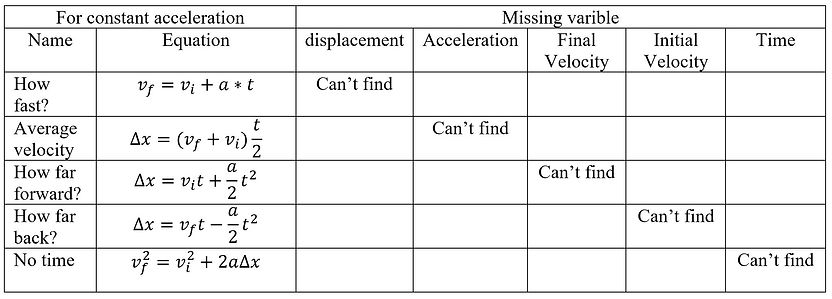top of page

## Kinematics

This is the most basic method to use an equation of motion (EOM) from free body diagrams to predict the motion of a body.

First of all, we need to define what acceleration, velocity, and position are.

The position is the location of the body (object) measured with the displacement relative to a reference frame; this reference point can be moving or fixed in space, but as long as the reference frame is not rotating (see this later chapter) the rest of the math works, even if the object is rotating. The displacement is generally defined with a vector, displacement in the x and y directions.

The velocity is the rate of change of the position vector, this can be expressed as the average velocity, change in position over the change in time (see below), or the instantaneous velocity, aka the derivative of position (make delta-T really small). you may have heard the phrase "velocity has magnitude and direction" and that ties into the definition of a vector. Velocity can be positive or negative, generally, pos for up and right, and neg for left and down. There will be a component of velocity in each direction; the speed is the magnitude of the vector or the Pythagorean sum of each component, so the speed is always a positive value.

Acceleration is the rate of change of the velocity vector, so acceleration is to velocity as what velocity is to position. This also defines acceleration as the 2nd derivative of position. Some more advanced students may recognize this as a 2nd order differential equation, and this makes solutions for systems where acceleration changes with respect to position hard (see periodic motion). However, if acceleration only varies with time, an integral can be used to find an analytical solution. If acceleration is a constant (ie free fall) the equations below can be used if time is a variable.The v_avg equation should be end-minus-start to be more correct, but the start/end and f/i order does not matter as long as the top and bottom of the equation have the same order, but final - initial is considered standard.

If position is not cared about, the velocity_(t) equation can be used, or the average acceleration equation (below) works whether acceleration is constant or changing. However, if the time is not cared about (by this I mean, not given, and not requested; although time could be used in-between steps.), use a modified version of the work-energy equation, also shown below.So, the kinematic equations can be summarized with these 5, with what variables are included and which are not cared about noted in the chart.

Solving for the unknown variable is up to you, however, note that equations 3 and 4 are the same thing, just 4 stats at the end conditions and outputs the displacement backward as a positive number. If delta t is unknown, the quadratic formula must be used. (note that the "plus or minus" yields 2 results, and one time is usually negative, and only one will make sense in the context of your problem.)

Equation 5 does not tell you the direction of the final velocity because the square of a negative number is positive, so the square root is indeterminately pos or neg; use your judgment in the context of the problem.bottom of page